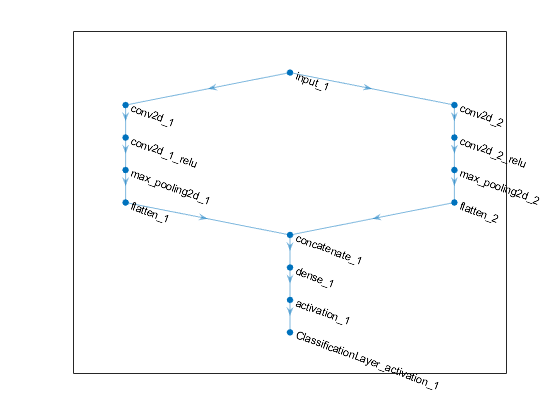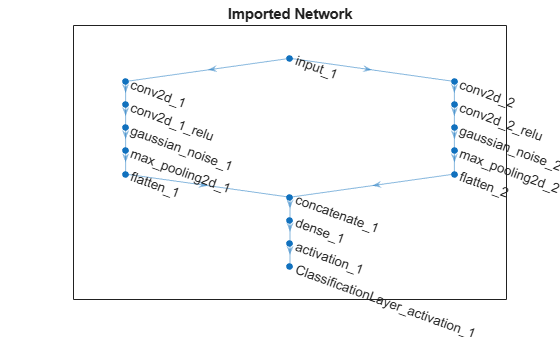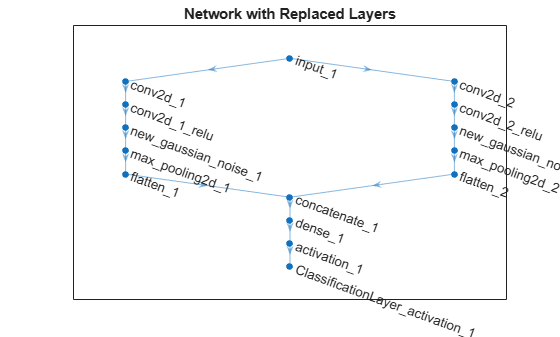# importKerasLayers

Import layers from Keras network

## Syntax

``layers = importKerasLayers(modelfile)``
``layers = importKerasLayers(modelfile,Name,Value)``

## Description

example

````layers = importKerasLayers(modelfile)` imports the layers of a TensorFlow™-Keras network from a model file. The function returns the layers defined in the HDF5 (`.h5`) or JSON (`.json`) file given by the file name `modelfile`.This function requires the Deep Learning Toolbox™ Importer for TensorFlow-Keras Models support package. If this support package is not installed, then the function provides a download link.```

example

````layers = importKerasLayers(modelfile,Name,Value)` imports the layers from a TensorFlow-Keras network with additional options specified by one or more name-value pair arguments.For example, `importKerasLayers(modelfile,'ImportWeights',true)` imports the network layers and the weights from the model file `modelfile`.```

## Examples

collapse all

Download and install the Deep Learning Toolbox Importer for TensorFlow-Keras Models support package.

Type `importKerasLayers` at the command line.

```importKerasLayers ```

If the Deep Learning Toolbox Importer for TensorFlow-Keras Models support package is not installed, then the function provides a link to the required support package in the Add-On Explorer. To install the support package, click the link, and then click Install. Check that the installation is successful by importing the layers from the model file `'digitsDAGnet.h5'` at the command line. If the required support package is installed, then the function returns a `LayerGraph` object.

```modelfile = 'digitsDAGnet.h5'; net = importKerasLayers(modelfile)```
```net = LayerGraph with properties: Layers: [13x1 nnet.cnn.layer.Layer] Connections: [13x2 table] InputNames: {'input_1'} OutputNames: {'ClassificationLayer_activation_1'} ```

Import the network layers from the model file `digitsDAGnet.h5`.

```modelfile = 'digitsDAGnet.h5'; layers = importKerasLayers(modelfile) ```
```layers = LayerGraph with properties: Layers: [13x1 nnet.cnn.layer.Layer] Connections: [13x2 table] InputNames: {'input_1'} OutputNames: {'ClassificationLayer_activation_1'} ```

Plot the network architecture.

`plot(layers)`Specify the network file to import.

`modelfile = 'digitsDAGnet.h5';`

Import network layers.

`layers = importKerasLayers(modelfile)`
```layers = LayerGraph with properties: Layers: [13x1 nnet.cnn.layer.Layer] Connections: [13x2 table] InputNames: {'input_1'} OutputNames: {'ClassificationLayer_activation_1'} ```

Load a data set for training a classifier to recognize new digits.

```folder = fullfile(toolboxdir('nnet'),'nndemos','nndatasets','DigitDataset'); imds = imageDatastore(folder, ... 'IncludeSubfolders',true, ... 'LabelSource','foldernames');```

Partition the dataset into training and test sets.

```numTrainFiles = 750; [imdsTrain,imdsTest] = splitEachLabel(imds,numTrainFiles,'randomize');```

Set the training options.

```options = trainingOptions('sgdm', ... 'MaxEpochs',10, ... 'InitialLearnRate',0.001);```

Train network using training data.

`net = trainNetwork(imdsTrain,layers,options);`
```Training on single CPU. |========================================================================================| | Epoch | Iteration | Time Elapsed | Mini-batch | Mini-batch | Base Learning | | | | (hh:mm:ss) | Accuracy | Loss | Rate | |========================================================================================| | 1 | 1 | 00:00:00 | 15.62% | 12.6982 | 0.0010 | | 1 | 50 | 00:00:09 | 63.28% | 1.2109 | 0.0010 | | 2 | 100 | 00:00:19 | 85.16% | 0.4193 | 0.0010 | | 3 | 150 | 00:00:30 | 96.88% | 0.1749 | 0.0010 | | 4 | 200 | 00:00:42 | 99.22% | 0.0457 | 0.0010 | | 5 | 250 | 00:00:53 | 100.00% | 0.0374 | 0.0010 | | 6 | 300 | 00:01:04 | 96.88% | 0.1223 | 0.0010 | | 7 | 350 | 00:01:15 | 100.00% | 0.0087 | 0.0010 | | 7 | 400 | 00:01:25 | 100.00% | 0.0166 | 0.0010 | | 8 | 450 | 00:01:35 | 100.00% | 0.0098 | 0.0010 | | 9 | 500 | 00:01:47 | 100.00% | 0.0047 | 0.0010 | | 10 | 550 | 00:01:58 | 100.00% | 0.0031 | 0.0010 | | 10 | 580 | 00:02:04 | 100.00% | 0.0059 | 0.0010 | |========================================================================================| ```

Run the trained network on the test set that was not used to train the network and predict the image labels (digits).

```YPred = classify(net,imdsTest); YTest = imdsTest.Labels;```

Calculate the accuracy.

`accuracy = sum(YPred == YTest)/numel(YTest)`
```accuracy = 0.9856 ```

Specify the network file to import layers and weights from.

`modelfile = 'digitsDAGnet.h5';`

Import the network architecture and weights from the files you specified. To import the layer weights, specify `'ImportWeights'` to be `true`. The function also imports the layers with their weights from the same HDF5 file.

`layers = importKerasLayers(modelfile,'ImportWeights',true)`
```layers = LayerGraph with properties: Layers: [13x1 nnet.cnn.layer.Layer] Connections: [13x2 table] InputNames: {'input_1'} OutputNames: {'ClassificationLayer_activation_1'} ```

View the size of the weights in the second layer.

```weights = layers.Layers(2).Weights; size(weights)```
```ans = 1×4 7 7 1 20 ```

The function has imported the weights so the layer weights are non-empty.

Specify the network file to import layers from and the file containing weights.

```modelfile = 'digitsDAGnet.json'; weights = 'digitsDAGnet.weights.h5';```

Import the network architecture and weights from the files you specified. The .json file does not include an output layer. Specify the output layer, so that importKerasLayers adds an output layer at the end of the networks architecture.

```layers = importKerasLayers(modelfile, ... 'ImportWeights',true, ... 'WeightFile',weights, ... 'OutputLayerType','classification')```
```layers = LayerGraph with properties: Layers: [13x1 nnet.cnn.layer.Layer] Connections: [13x2 table] InputNames: {'input_1'} OutputNames: {'ClassificationLayer_activation_1'} ```

This example shows how to import the layers from a pretrained Keras network, replace the unsupported layers with custom layers, and assemble the layers into a network ready for prediction.

Import Keras Network

Import the layers from a Keras network model. The network in `'digitsDAGnetwithnoise.h5'` classifies images of digits.

```filename = 'digitsDAGnetwithnoise.h5'; lgraph = importKerasLayers(filename,'ImportWeights',true);```
```Warning: Unable to import some Keras layers, because they are not supported by the Deep Learning Toolbox. They have been replaced by placeholder layers. To find these layers, call the function findPlaceholderLayers on the returned object. ```

The Keras network contains some layers that are not supported by Deep Learning Toolbox. The `importKerasLayers` function displays a warning and replaces the unsupported layers with placeholder layers.

Plot the layer graph using `plot`.

```figure plot(lgraph) title("Imported Network")```Replace Placeholder Layers

To replace the placeholder layers, first identify the names of the layers to replace. Find the placeholder layers using `findPlaceholderLayers`.

`placeholderLayers = findPlaceholderLayers(lgraph)`
```placeholderLayers = 2x1 PlaceholderLayer array with layers: 1 'gaussian_noise_1' PLACEHOLDER LAYER Placeholder for 'GaussianNoise' Keras layer 2 'gaussian_noise_2' PLACEHOLDER LAYER Placeholder for 'GaussianNoise' Keras layer ```

Display the Keras configurations of these layers.

`placeholderLayers.KerasConfiguration`
```ans = struct with fields: trainable: 1 name: 'gaussian_noise_1' stddev: 1.5000 ```
```ans = struct with fields: trainable: 1 name: 'gaussian_noise_2' stddev: 0.7000 ```

Define a custom Gaussian noise layer. To create this layer, save the file `gaussianNoiseLayer.m` in the current folder. Then, create two Gaussian noise layers with the same configurations as the imported Keras layers.

```gnLayer1 = gaussianNoiseLayer(1.5,'new_gaussian_noise_1'); gnLayer2 = gaussianNoiseLayer(0.7,'new_gaussian_noise_2');```

Replace the placeholder layers with the custom layers using `replaceLayer`.

```lgraph = replaceLayer(lgraph,'gaussian_noise_1',gnLayer1); lgraph = replaceLayer(lgraph,'gaussian_noise_2',gnLayer2);```

Plot the updated layer graph using `plot`.

```figure plot(lgraph) title("Network with Replaced Layers")```Specify Class Names

If the imported classification layer does not contain the classes, then you must specify these before prediction. If you do not specify the classes, then the software automatically sets the classes to `1`, `2`, ..., `N`, where `N` is the number of classes.

Find the index of the classification layer by viewing the `Layers` property of the layer graph.

`lgraph.Layers`
```ans = 15x1 Layer array with layers: 1 'input_1' Image Input 28x28x1 images 2 'conv2d_1' Convolution 20 7x7x1 convolutions with stride [1 1] and padding 'same' 3 'conv2d_1_relu' ReLU ReLU 4 'conv2d_2' Convolution 20 3x3x1 convolutions with stride [1 1] and padding 'same' 5 'conv2d_2_relu' ReLU ReLU 6 'new_gaussian_noise_1' Gaussian Noise Gaussian noise with standard deviation 1.5 7 'new_gaussian_noise_2' Gaussian Noise Gaussian noise with standard deviation 0.7 8 'max_pooling2d_1' Max Pooling 2x2 max pooling with stride [2 2] and padding 'same' 9 'max_pooling2d_2' Max Pooling 2x2 max pooling with stride [2 2] and padding 'same' 10 'flatten_1' Keras Flatten Flatten activations into 1-D assuming C-style (row-major) order 11 'flatten_2' Keras Flatten Flatten activations into 1-D assuming C-style (row-major) order 12 'concatenate_1' Depth concatenation Depth concatenation of 2 inputs 13 'dense_1' Fully Connected 10 fully connected layer 14 'activation_1' Softmax softmax 15 'ClassificationLayer_activation_1' Classification Output crossentropyex ```

The classification layer has the name `'ClassificationLayer_activation_1'`. View the classification layer and check the `Classes` property.

`cLayer = lgraph.Layers(end)`
```cLayer = ClassificationOutputLayer with properties: Name: 'ClassificationLayer_activation_1' Classes: 'auto' OutputSize: 'auto' Hyperparameters LossFunction: 'crossentropyex' ```

Because the `Classes` property of the layer is `'auto'`, you must specify the classes manually. Set the classes to `0`, `1`, ..., `9`, and then replace the imported classification layer with the new one.

`cLayer.Classes = string(0:9)`
```cLayer = ClassificationOutputLayer with properties: Name: 'ClassificationLayer_activation_1' Classes: [0 1 2 3 4 5 6 7 8 9] OutputSize: 10 Hyperparameters LossFunction: 'crossentropyex' ```
`lgraph = replaceLayer(lgraph,'ClassificationLayer_activation_1',cLayer);`

Assemble Network

Assemble the layer graph using `assembleNetwork`. The function returns a `DAGNetwork` object that is ready to use for prediction.

`net = assembleNetwork(lgraph)`
```net = DAGNetwork with properties: Layers: [15x1 nnet.cnn.layer.Layer] Connections: [15x2 table] InputNames: {'input_1'} OutputNames: {'ClassificationLayer_activation_1'} ```

Import layers from a Keras network that has parametric rectified linear unit (PReLU) layers.

A PReLU layer performs a threshold operation, where for each channel, any input value less than zero is multiplied by a scalar. The PReLU operation is given by

`$\mathit{f}\left({\mathit{x}}_{\mathit{i}}\right)=\left\{\begin{array}{ll}{\mathit{x}}_{\mathit{i}}& \mathrm{if}\text{\hspace{0.17em}}{\mathit{x}}_{\mathit{i}}>0\\ {\mathit{a}}_{\mathit{i}}{\mathit{x}}_{\mathit{i}}& \mathrm{if}\text{\hspace{0.17em}}{\mathit{x}}_{\mathit{i}}\le 0\end{array}$`

where ${\mathit{x}}_{\mathit{i}}$ is the input of the nonlinear activation $\mathit{f}$ on channel $i$, and ${a}_{i}$ is the scaling parameter controlling the slope of the negative part. The subscript $i$ in ${a}_{i}$ indicates that the parameter can be a vector and the nonlinear activation can vary on different channels.

`importKerasNetwork` and `importKerasLayers` can import a network that includes PReLU layers. These functions support both scalar-valued and vector-valued scaling parameters. If a scaling parameter is a vector, then the functions replace the vector with the average of the vector elements. You can modify a PReLU layer to have a vector-valued scaling parameter after import.

Specify the network file to import.

`modelfile = 'digitsDAGnetwithPReLU.h5';`

`digitsDAGnetwithPReLU` includes two PReLU layers. One has a scalar-valued scaling parameter, and the other has a vector-valued scaling parameter.

Import the network architecture and weights from `modelfile`.

`layers = importKerasLayers(modelfile,'ImportWeights',true);`
```Warning: Layer 'p_re_lu_1' is a PReLU layer with a vector-valued parameter. The function replaces the parameter with the average of the vector elements. You can change the parameter back to a vector after import. ```

The `importKerasLayers` function displays a warning for the PReLu layer `p_re_lu_1`. The function replaces the vector-valued scaling parameter of `p_re_lu_1` with the average of the vector elements. You can change the parameter back to a vector. First, find the index of the PReLU layer by viewing the `Layers` property.

`layers.Layers`
```ans = 13x1 Layer array with layers: 1 'input_1' Image Input 28x28x1 images 2 'conv2d_1' Convolution 20 7x7x1 convolutions with stride [1 1] and padding 'same' 3 'conv2d_2' Convolution 20 3x3x1 convolutions with stride [1 1] and padding 'same' 4 'p_re_lu_1' PReLU PReLU layer 5 'p_re_lu_2' PReLU PReLU layer 6 'max_pooling2d_1' Max Pooling 2x2 max pooling with stride [2 2] and padding 'same' 7 'max_pooling2d_2' Max Pooling 2x2 max pooling with stride [2 2] and padding 'same' 8 'flatten_1' Keras Flatten Flatten activations into 1-D assuming C-style (row-major) order 9 'flatten_2' Keras Flatten Flatten activations into 1-D assuming C-style (row-major) order 10 'concatenate_1' Depth concatenation Depth concatenation of 2 inputs 11 'dense_1' Fully Connected 10 fully connected layer 12 'dense_1_softmax' Softmax softmax 13 'ClassificationLayer_dense_1' Classification Output crossentropyex ```

`layers` has two PReLU layers. Extract the fourth layer `p_re_lu_1`, which originally had a vector-valued scaling parameter for a channel dimension.

`tempLayer = layers.Layers(4)`
```tempLayer = PreluLayer with properties: Name: 'p_re_lu_1' RawAlpha: [20x1 single] Learnable Parameters Alpha: 0.0044 Show all properties ```

The `RawAlpha` property contains the vector-valued scaling parameter, and the `Alpha` property contains a scalar that is an element average of the vector values. Reshape `RawAlpha` to place the vector values in the third dimension, which corresponds to the channel dimension. Then, replace `Alpha` with the reshaped `RawAlpha` values.

`tempLayer.Alpha = reshape(tempLayer.RawAlpha,[1,1,numel(tempLayer.RawAlpha)])`
```tempLayer = PreluLayer with properties: Name: 'p_re_lu_1' RawAlpha: [20x1 single] Learnable Parameters Alpha: [1x1x20 single] Show all properties ```

Replace the `p_re_lu_1` layer in `layers` with `tempLayer`.

```layers = replaceLayer(layers,'p_re_lu_1', tempLayer); layers.Layers(4)```
```ans = PreluLayer with properties: Name: 'p_re_lu_1' RawAlpha: [20x1 single] Learnable Parameters Alpha: [1x1x20 single] Show all properties ```

Now the `p_re_lu_1` layer has a vector-valued scaling parameter.

## Input Arguments

collapse all

Name of the model file containing the network architecture, and possibly the weights, specified as a character vector or a string scalar. The file must be in the current folder, in a folder on the MATLAB® path, or you must include a full or relative path to the file.

If `modelfile` includes

• The network architecture and weights, then it must be in HDF5 (`.h5`) format.

• Only the network architecture, then it can be in HDF5 or JSON (`.json`) format.

If `modelfile` includes only the network architecture, then you can optionally supply the weights using the `'ImportWeights'` and `'WeightFile'` name-value pair arguments. If you supply the weights, then the weights file must be in HDF5 format.

Example: `'digitsnet.h5'`

Data Types: `char` | `string`

### Name-Value Pair Arguments

Specify optional comma-separated pairs of `Name,Value` arguments. `Name` is the argument name and `Value` is the corresponding value. `Name` must appear inside quotes. You can specify several name and value pair arguments in any order as `Name1,Value1,...,NameN,ValueN`.

Example: `importKerasLayers(modelfile,'OutputLayerType','classification')` imports the network layers from the model file `modelfile` and adds an output layer for a classification problem at the end of the Keras layers.

Type of the output layer that the function appends to the end of the imported network architecture when `modelfile` does not specify a loss function, specified as `'classification'`, `'regression'`, or `'pixelclassification'`. Appending a `pixelClassificationLayer` (Computer Vision Toolbox) object requires Computer Vision Toolbox™.

If a network in `modelfile` has multiple outputs, then you cannot specify the output layer types using this argument. `importKerasLayers` inserts placeholder layers for the outputs. After importing, you can find and replace the placeholder layers by using `findPlaceholderLayers` and `replaceLayer`, respectively.

Example: `'OutputLayerType','regression'`

Size of the input images for the network, specified as a vector of two or three numerical values corresponding to `[height,width]` for grayscale images and `[height,width,channels]` for color images, respectively. The network uses this information when the `modelfile` does not specify the input size.

If a network in `modelfile` has multiple inputs, then you cannot specify the input sizes using this argument. `importKerasLayers` inserts placeholder layers for the inputs. After importing, you can find and replace the placeholder layers by using `findPlaceholderLayers` and `replaceLayer`, respectively.

Example: `'ImageInputSize',[28 28]`

Indicator to import weights as well as the network architecture, specified as either `false` or `true`.

• If `'ImportWeights'` is `true` and `modelfile` includes the weights, then `importKerasLayers` imports the weights from `modelfile`, which must have HDF5 (`.h5`) format.

• If `'ImportWeights'` is `true` and `modelfile` does not include the weights, then you must specify a separate file that includes weights, using the `'WeightFile'` name-value pair argument.

Example: `'ImportWeights',true`

Data Types: `logical`

Weight file name, from which to import weights when `modelfile` does not include weights, specified as a character vector or a string scalar. To use this name-value pair argument, you also must set `'ImportWeights'` to `true`.

Weight file must be in the current folder, in a folder on the MATLAB path, or you must include a full or relative path to the file.

Example: `'WeightFile','weights.h5'`

Data Types: `char` | `string`

## Output Arguments

collapse all

Network architecture, returned as a `Layer` array object when the Keras network is of type `Sequential`, or returned as a `LayerGraph` object when the Keras network is of type `Model`.

## Tips

 Keras: The Python Deep Learning library. https://keras.io.